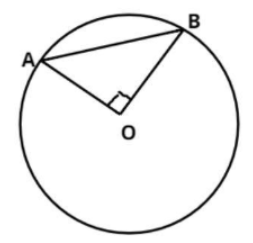Courses
Courses for Kids
Free study material
Offline Centres
MoreLast updated date: 01st Dec 2023
Total views: 284.8k
Views today: 6.84k

# A chord of a circle of radius 10 cm subtends a right angle at its center. The length of chord (in cm) is$a.{\text{ 5}}\sqrt 2 \\ b.{\text{ 10}}\sqrt 2 \\ c.{\text{ }}\dfrac{5}{{\sqrt 2 }} \\ d.{\text{ 10}}\sqrt 3 \\$Verified
284.8k+ views
Hint – Length of the lines joining the end points of chords of a circle to the centre of a circle are radius , Thus the triangle formed here is the right angle isosceles triangle (given) . Clearly PGT can be used here .The pictorial representation of the given problem is shown above.
Let AB be the chord of the circle.
O is the center of the circle, chord AB subtends a right angle at its center.
$\therefore \angle AOB = {90^0}$
OA = OB = 10 cm radius of the circle.
Now we have to find out the length of the chord AB.
So, apply Pythagoras theorem in triangle AOB
$\Rightarrow {\left( {{\text{Hypotenuse}}} \right)^2} = {\left( {{\text{Base}}} \right)^2} + {\left( {{\text{Perpendicular}}} \right)^2} \\ \Rightarrow {\left( {AB} \right)^2} = {\left( {OB} \right)^2} + {\left( {OA} \right)^2} \\ \Rightarrow {\left( {AB} \right)^2} = {10^2} + {10^2} = 100 + 100 = 200 \\ \Rightarrow AB = \sqrt {200} = 10\sqrt 2 {\text{ cm}} \\$
So, the length of the chord is $10\sqrt 2 {\text{ cm}}$.
Hence, option (b) is correct.

Note – In such types of questions first draw the pictorial representation of the given problem as above then always remember the property of Pythagoras Theorem which is stated above, then using this property calculate the length of the chord which is the required answer.